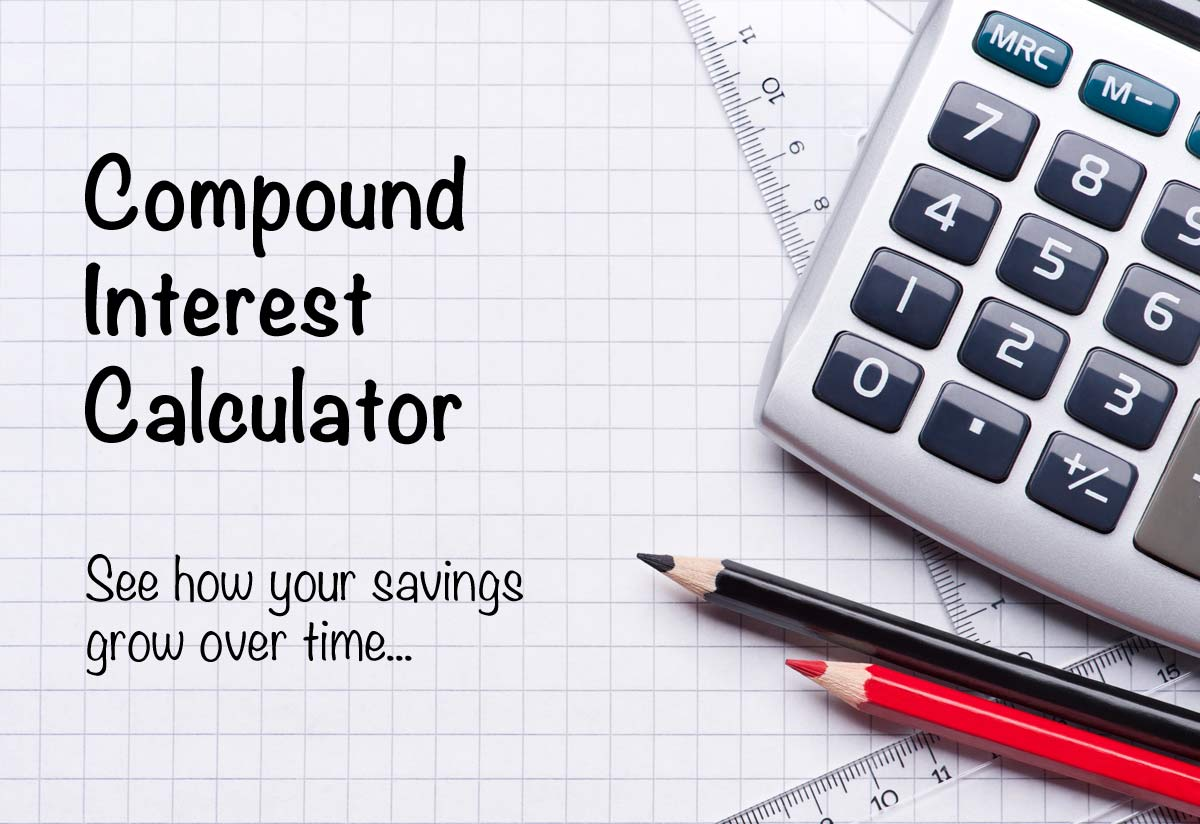# How to calculate the monthly payable amount on loan using an interest rate calculator?494 Views

When you take an instant personal loan online, it becomes very important for you to calculate the cost of the loan. The total cost and the monthly amount payable after taking the loan are the most important aspects of borrowing. To make an easy calculation and choose the right amount of EMI every month, you just need an EMI or loan calculator. It is available online and makes it easy for you to calculate and manage all your EMI payments and find the right borrowing amount. Before you borrow and use an EMI calculator, you must have all the knowledge of how to use it and why you are using it. Clix personal loan calculator is the best way to calculate your EMI and this helps you to choose the right amount.

## What is an interest rate calculator?

When you take a personal loan, you must use an EMI calculator to make sure you calculate your monthly payment and calculate the interest rate in the best way. When you decide to use a calculator, it will be easy for you to calculate and avoid all kinds of miscalculations. There are various ways in which you will need an interest rate calculator that is available on the online website of Clix Capital. An EMI calculator is a special way to handle all your needs and emergencies in the right way. This will keep you posted on calculating your monthly instalments the right way. You can avoid all the miscalculations and make way for easy calculation.

## What does an EMI denote?

An EMI is an equated monthly instalment that is the scheme of repayment. It is not possible for a borrower to pay off the entire home loan at once, so the amount is broken down into small amounts every month. The EMI has the principal loan amount and the rate of interest in it. If you choose the right EMI calculator, then there will not be any hurdles. To get a low EMI, you need to focus on things like eligibility criteria, low rates of interest, and the right tenure. If you are finding it difficult, you can use an EMI calculator that is meant for calculating the EMI. There is a formula to calculate the EMI and that makes it very easy. Find out more about EMI and its calculation.

## Elements of the EMI calculator

Principal amount: This is the amount that a borrower takes from the loan provider. This is the actual debt amount. If you borrow a high principal amount, the EMI will be high.

Tenure: This is the time period of the home loan. You can choose the tenure according to your repayment capacity. If you want a low EMI, you should choose a long-term loan.

Rate of interest: A rate of interest is charged by the loan provider on the amount you borrow. It is a factor that needs to be kept low to keep your EMI affordable.

## EMI = principal amount plus interest rate/term in months

When you calculate these three in this formula, you will get your EMI. Use a calculator to make the calculations accurate and easy. You need to add the principal loan amount to the rate of interest and then divide it by the number of months in the loan tenure. When you divide, you get your EMI. If you find the EMI to be high, you can make changes by increasing the tenure, settling for a low rate of interest, or decreasing the principal loan amount. This way, you will find the right EMI for your personal loan without making it a burden.

## How to choose the right EMI using a calculator?

Income: Based on your income, you need to decide your EMI and monthly payment. When you consider your income, you will be able to choose the right monthly amount that is payable every month. An EMI will help in understanding the right amount that you are capable of paying every month. Your income and EMI should be in sync so that you can get the best experience. This will help you with the right experience and there will not be any burden or debt default.

Expenses and obligations: When deciding on an EMI, you must consider the appropriate amount of obligations and fixed expenses that you have each month.Consider all the expenses and emergencies so that you choose the right EMI with the calculator that helps you quickly decide and compute your EMI amount every month. Understand all your spending habits.

Loan amount: EMI calculations will depend upon the loan amount. If your loan amount is low, you can easily go for a short-term loan. This will help you cover all the aspects of borrowing without any issues. For a high loan amount, you need to make sure you will get the right EMI amount by using a calculator.

## Finishing up

The personal loan apply online process of an instant personal loan online will help you with a smooth and affordable borrowing experience. Use a personal loan calculator so that you can find the right way to borrow.